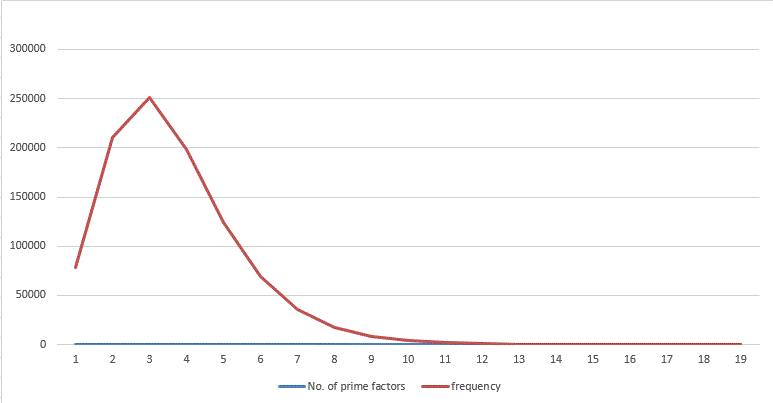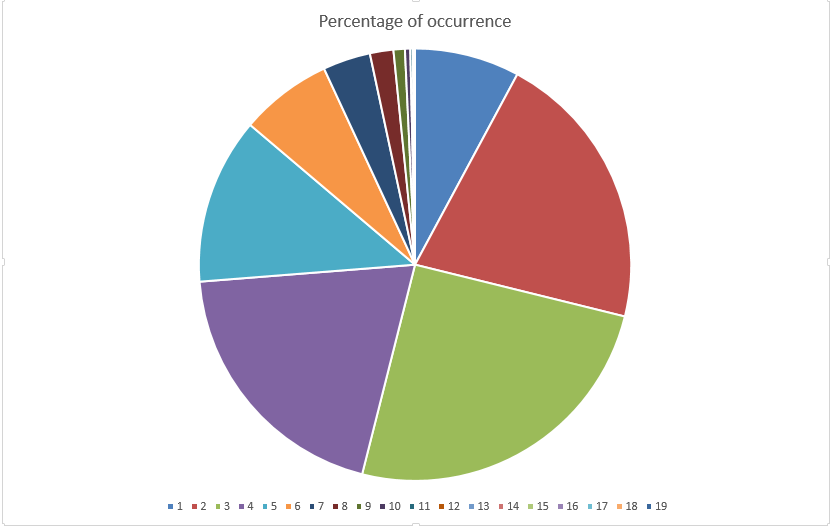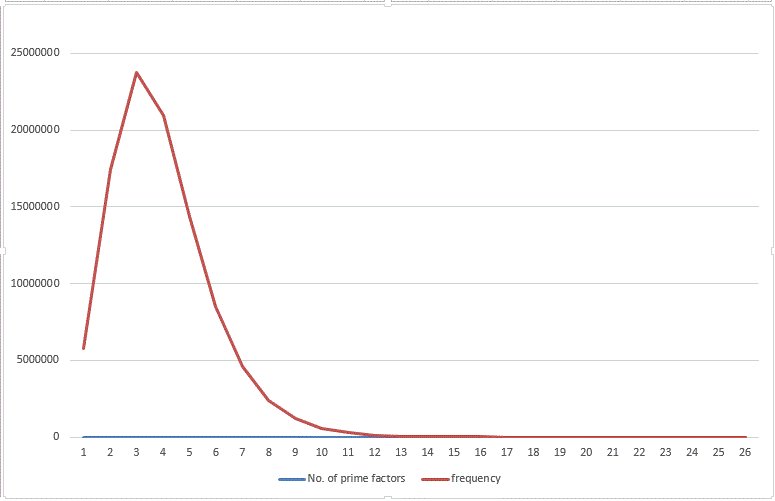# Analysis page (Most common Number of prime factors)

All the analysis made from the outputs of participants will be shown here.

• Graph of Prime factor distribution from $0$ to $10^6$:• Graph of Number of prime factors occurrence percentage (till $10^6$):• Graph of Prime factor distribution from $0$ to $10^8$:Note :

• If you have another idea (rather than line graph) of representing the data then please share!

• Thanks to Páll Márton to submit answer till $10^8$.Note by Zakir Husain
12 months ago

This discussion board is a place to discuss our Daily Challenges and the math and science related to those challenges. Explanations are more than just a solution — they should explain the steps and thinking strategies that you used to obtain the solution. Comments should further the discussion of math and science.

When posting on Brilliant:

• Use the emojis to react to an explanation, whether you're congratulating a job well done , or just really confused .
• Ask specific questions about the challenge or the steps in somebody's explanation. Well-posed questions can add a lot to the discussion, but posting "I don't understand!" doesn't help anyone.
• Try to contribute something new to the discussion, whether it is an extension, generalization or other idea related to the challenge.

MarkdownAppears as
*italics* or _italics_ italics
**bold** or __bold__ bold
- bulleted- list
• bulleted
• list
1. numbered2. list
1. numbered
2. list
Note: you must add a full line of space before and after lists for them to show up correctly
paragraph 1paragraph 2

paragraph 1

paragraph 2

[example link](https://brilliant.org)example link
> This is a quote
This is a quote
    # I indented these lines
# 4 spaces, and now they show
# up as a code block.

print "hello world"
# I indented these lines
# 4 spaces, and now they show
# up as a code block.

print "hello world"
MathAppears as
Remember to wrap math in $$ ... $$ or $ ... $ to ensure proper formatting.
2 \times 3 $2 \times 3$
2^{34} $2^{34}$
a_{i-1} $a_{i-1}$
\frac{2}{3} $\frac{2}{3}$
\sqrt{2} $\sqrt{2}$
\sum_{i=1}^3 $\sum_{i=1}^3$
\sin \theta $\sin \theta$
\boxed{123} $\boxed{123}$

Sort by:

@Zakir Husain I think Pie chart will be better as it will help visualise more clearly.

- 12 months ago

Sure!

- 12 months ago

It seems that till $10^6$ numbers with number of prime factors - $2,3,4$ are most common. Also highly composite number are more rare than primes

- 12 months ago

Reminds me of $\dfrac{1}{(x-k)^n}$...

- 12 months ago

What? I didn't understood, what you meant?

- 12 months ago

x=number of prime factors, k & n are unknown constants, the output of the function is the number of number that have x prime factors.

- 12 months ago

Matches the graph

- 12 months ago

- 12 months ago

Except for $x < 2$ I guess?

- 12 months ago

Looking great @Zakir Husain ! Could you share the actual numbers as well?

- 12 months ago

$\Large{Announcement:}$ New plan is here, everything is nearly changed now

- 4 months ago